## A bit of Mathematics, Physics and Magic

by Dr. Tao Chen and Dr. Roman Senkov

### Foreword

Seeing a master at work is always mesmerizing, as if they are performing magic in front of our very eyes. It doesn't matter if we are looking at a culinary chef, a musician, an athlete, or a mathematician. It can be a source of great inspiration. Despite understanding that this magic that we see actually originates from knowledge and years of practice, we're still inspired to pursue their magic ourselves.

In this short article, we will try to demonstrate a few simple, but beautiful mathematical “tricks” and methods, that will allows us to solve quite challenging problems with minimal effort (in this case the problems only appear to be challenging - otherwise they would not have such simple solutions or the hardest part of the solution is somehow hidden). At the end of the article we show how these methods can be applied to some real and not-very-real physics problems.

We hope you'll enjoy the reading and the magic behind equations and formulae. Please feel free to contact us with any questions and, perhaps, solutions.

Tao Chen (tchen@lagcc.cuny.edu),
Roman Senkov (rsenkov@lagcc.cuny.edu)

### 1. Geometric and Other Series

A series is the sum of infinitely many terms. Usually, adding an infinite amount of terms is a daunting task. Luckily, if these terms have a pattern or they can be derived from a previously derived series the problem becomes manageable. For example, to find \begin{equation}\tag{1} S_1(x) =1 + x + x^2 + x^3 + \cdots \end{equation} we can rearrange the sum as \begin{equation}\tag{2} S_1(x)= 1+x(1 + x + x^2 + x^3 + \cdots). \end{equation} We notice that the expression in the brackets is equal to $$S_1(x)$$, so we can rewrite the previous equation as \begin{equation}\tag{3} S_1(x)=1+x\cdot S_1(x) \end{equation} and therefore \begin{equation}\tag{4} S_1(x)=1 + x + x^2 + x^3 + \cdots = \frac{1}{1-x}. \end{equation} It has to be mentioned that these manipulations require certain conditions such as convergence to be true, that is, the sum of infinitely many terms to be valid (which has to be proven!). Without convergence, the above derivation is not valid. To see the problem try to find $$S_1(x)$$ for $$x=1$$ or $$x=2$$ (actually the sum in Eq.(1) exists only for $$|x| < 1$$ ).

The series in Eq.(1) is called a geometric series, but it also helps to find the sum of other series. For example, let \begin{equation}\tag{5} S_2(x) = x + \frac{x^2}{2} + \frac{x^3}{3} + \frac{x^4}{4}+ \cdots . \end{equation} Note that \begin{equation}\tag{6} \frac{d S_2(x)}{dx} = 1+x+x^2+x^3+\cdots = S_1(x) \end{equation} is a differential equation, $$S'_2(x)=S_1(x)$$. Therefore we can integrate it as \begin{equation}\tag{7} S_2(x)=\int \frac{1}{1-x}dx = - \ln (1-x). \end{equation} Using Eq.(7) we can demonstrate that the following series diverges logarithmically \begin{equation}\tag{8} 1 + \frac{1}{2} + \frac{1}{3} + \frac{1}{4}+ \cdots = \lim_{x \rightarrow 1} \left[- \ln(1-x)\right] = \infty . \end{equation} A similar technique could be applied to find the following famous series \begin{equation}\tag{9} S_3(x) = 1 + x + \frac{x^2}{2!} + \frac{x^3}{3!} + \cdots. \end{equation} We leave it to the readers: find $$S_3(x)$$ and prove that \begin{equation}\tag{10} e = 1 + 1 + \frac{1}{2!} + \frac{1}{3!} + \cdots, \end{equation}where $$e \approx 2.7182818\dots$$ is the Euler's number.

### 2. Continued Fractions

Continued fractions are written as fractions within fractions, which are added up in a special way, and which may go on for ever. In fact any fraction can be written as a continued fraction with finite length, such as 11/30 which can be written as \begin{equation} \cfrac{1}{2+\cfrac{2}{2+\cfrac{2}{2+\cfrac{2}{3}}}}; \end{equation} while an irrational number can be written as a continued fraction with infinite length. Similar to a series, continued fractions can be found if there is some pattern. For example, let \begin{equation}\tag{11} F_1 = 1+\cfrac{1}{1+\cfrac{1}{1+\cfrac{1}{1+\cfrac{1}{1+\dots}}}}. \end{equation} It is easy to see that $F_1=1+\frac{1}{F_1},$ or $$F_1$$ satisfies the equation $$x^2-x-1=0$$. Thus $$F_1=\frac{\sqrt{5}+1}{2}$$. What happened to the second root of the quadratic equation?

Can you find a more general continued fraction yourself? \begin{equation}\tag{12} F_2(x) = x+\cfrac{1}{x+\cfrac{1}{x+\cfrac{1}{x+\cfrac{1}{x+\dots}}}}. \end{equation} We can also find more complicated continued fractions, for example, like this one \begin{equation}\tag{13} F_3 = 1+\cfrac{1}{2+\cfrac{1}{1+\cfrac{1}{2+\cfrac{1}{1+\dots}}}}. \end{equation} Show that $$F_3$$ satisfies the following equation \begin{equation}\tag{14} F_3 = 1+\cfrac{1}{2+\cfrac{1}{F_3}} \end{equation} and find $$F_3$$. We leave the solution to diligent readers.

### 3. Nested Roots

A nested root is a radical expression that contains other radical expressions (nests). Similar to the series and continued fractions, we can also find the precise value of a nested root if it has a certain pattern. For example, let \begin{equation}\tag{15} R_1 = \sqrt{2+\sqrt{2+\sqrt{2+ \cdots}}} \end{equation} It is easy to see that $$R_1=\sqrt{2+R_1}$$. That is, $$R_1$$ satisfies the equation $$x^2-x-2=0$$. Thus $$R_2=2$$. Again, what will happen to the second root of the quadratic equation?

The readers can try to find the following three nested roots: \begin{equation}\tag{16} R_2(x) = \sqrt{x+\sqrt{x+\sqrt{x+ \cdots}}}, x\geq 0 \end{equation} \begin{equation}\tag{17} R_3 = \sqrt{2- \sqrt{2-\sqrt{2- \cdots}}}, \end{equation} and \begin{equation}\tag{18} R_4 = \sqrt{6+ \sqrt{6+\sqrt{6+ \cdots}}}. \end{equation}

### 4. Physics Examples

In this section we consider two physics-related problems. In the first example we calculate the effective resistance of an infinite series of resistors forming an electric circuit. The setup is slightly artificial, but still quite useful to consider, at least from theoretical point of view. The second example is a real-life problem from Quantum Field Theory (QFT). We discuss the idea behind the Schwinger-Dyson equations (SDEs) that involve summation of an infinite number of Feynman diagrams. Quantum field theory in general and SDEs in particular are quite complicated, so we will significantly simplify the problem considering it at a qualitative level.

#### 4.1 Infinite Series of Resistors

Consider the electric circuit shown in Fig. 1. One way to find the current through the battery $$V$$ is to replace the right-side part of the circuit with an equivalent resistance, $$R_{eq}$$.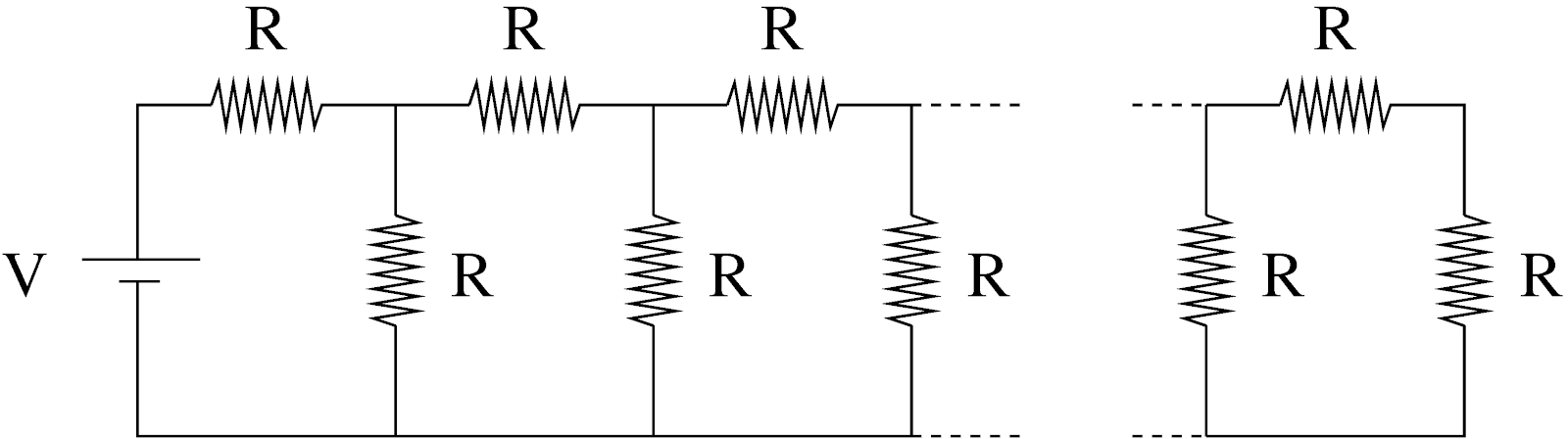Figure 1: Electric circuit with infinite number of identical resistors.

Equivalent resistance is an interesting and useful concept that is commonly used in electrical circuit theory. Figure 2 helps us to visualize the equivalent resistance for the given circuit.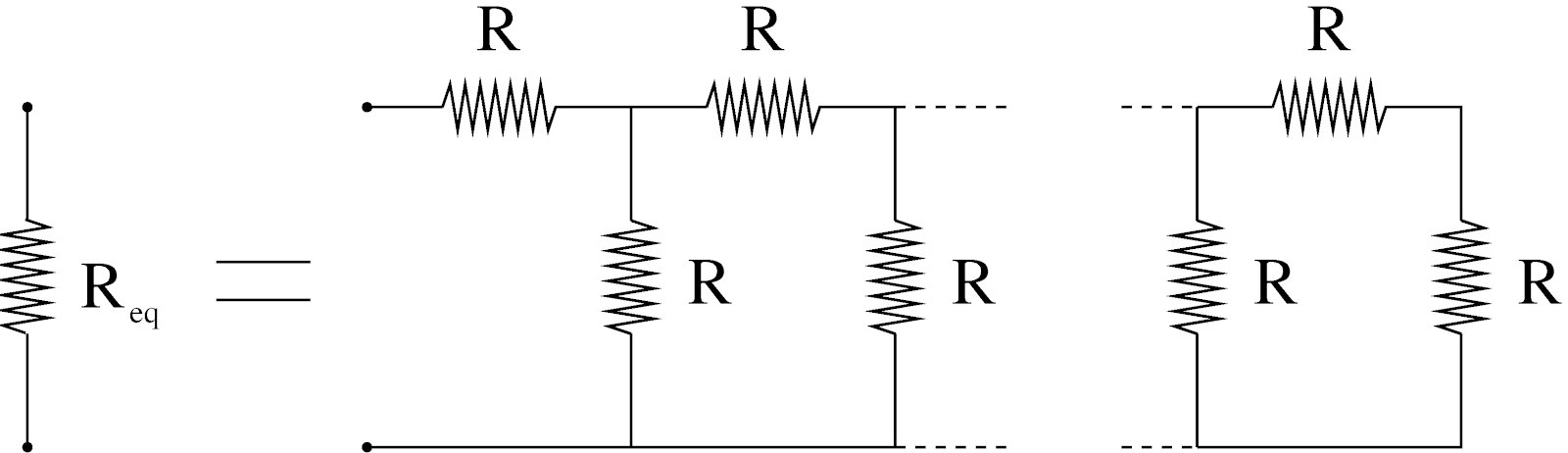Figure 2: The equivalent resistance.

Looking at Fig. 2 we notice that all of the resistors to the right from the two most left resistors (one is horizontal and one is vertical) form the same equivalent resistance $$R_{eq}$$. It is only true due to the fact that we have infinite number of resistors connected in the circuit. There is a certain similarity between this diagram and Eq.(2) that we had for the Geometric series. Thus we can proceed with the similar approach and set an equation for $$R_{eq}$$ similar to Eq.(3). Figure 3 presents this equation graphically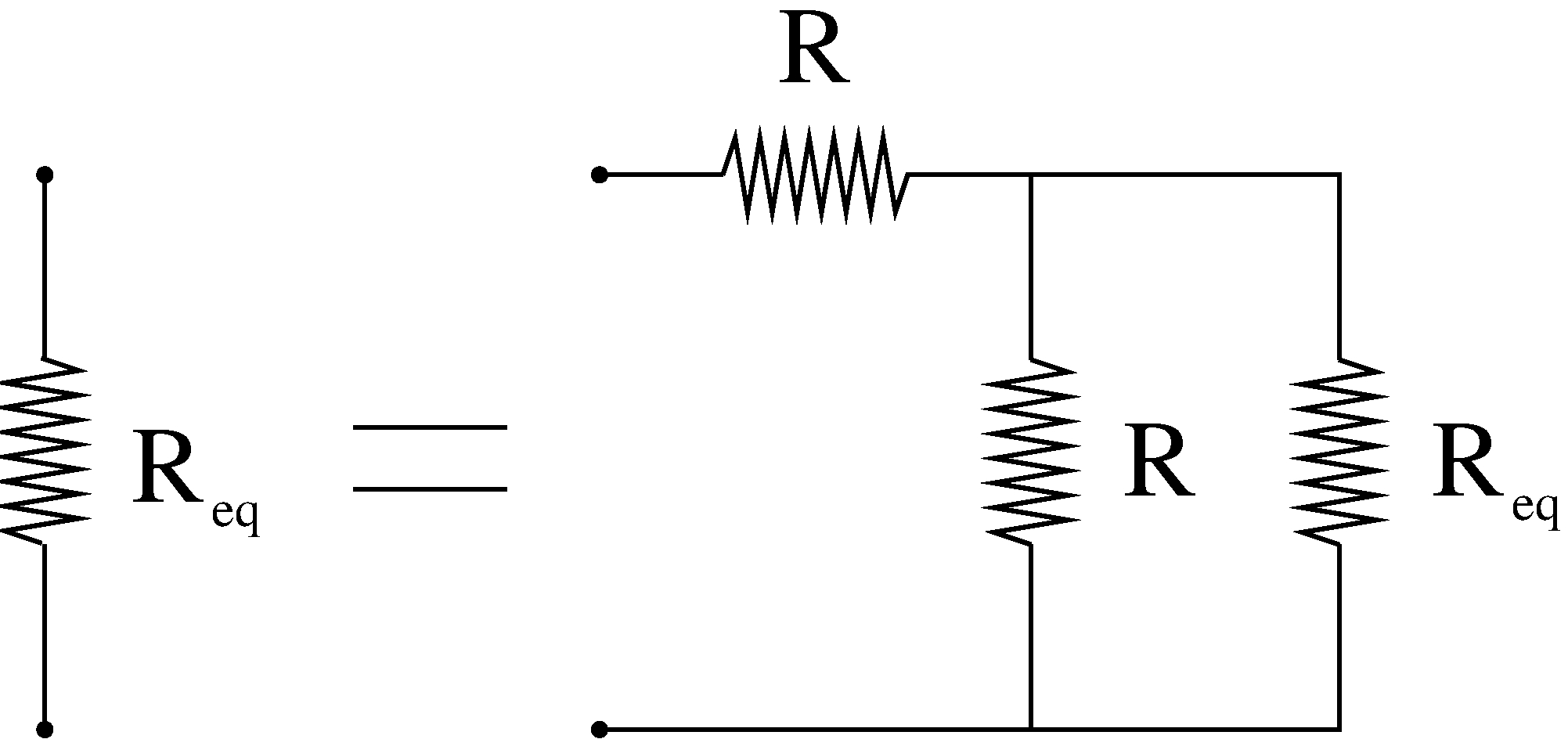Figure 3: The graphical representation of the equation (19) for the equivalent resistance.

while the analytical form of this equation is the following \begin{equation}\tag{19} R_{eq} = R + \frac{R R_{eq}}{R+R_{eq}}. \end{equation} Here we used certain well known rules for how to “add” resistances that are connected in parallel and series . The solution of the above equation gives us \begin{equation}\tag{20} R^2_{eq} - R^2 - R R_{eq} = 0 \mbox{ or } R_{eq} = \frac{1+\sqrt{5}}{2} R. \end{equation} If you liked and understood the idea, then try to find the equivalent capacitance for a similar circuit but with an infinite series of capacitors, see Fig. 4. Note that capacitors obey slightly different rules when connected in parallel/series - exactly opposite the behavior of resistors .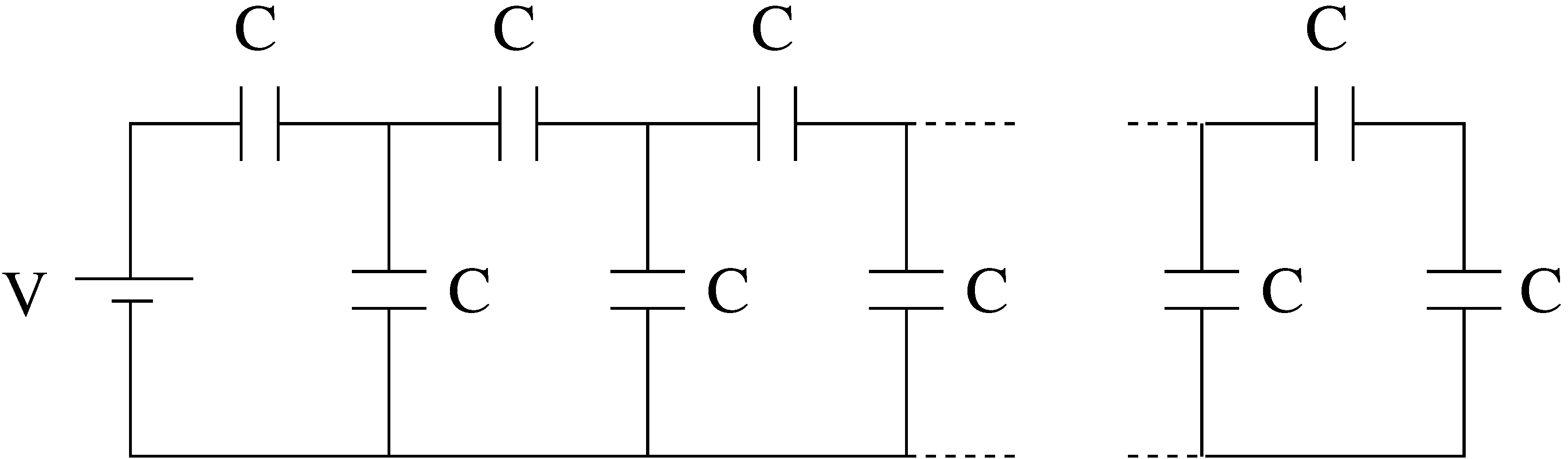Figure 4: A series of identical capacitors.

#### 4.2 The Idea behind Schwinger-Dyson Equations

In quantum theory we use wave function to describe the probability of finding the particle near certain position or Green's function $$G(x,y)$$ which has a similar meaning as the wave function but with the additional condition that initially the particle was at some position $$y$$.

The Dyson equation for the single-electron Green's function represented as an infinite series of Feynman diagrams as shown in Fig. 5. As we can see, there are infinite number of possibilities for the electron to reach point $$x$$ starting from point $$y$$, including free motion (the “bare electron” Green's function $$G_0(x,y)$$) and motion influenced by electromagnetic interaction (“dressed” with various photon exchanges).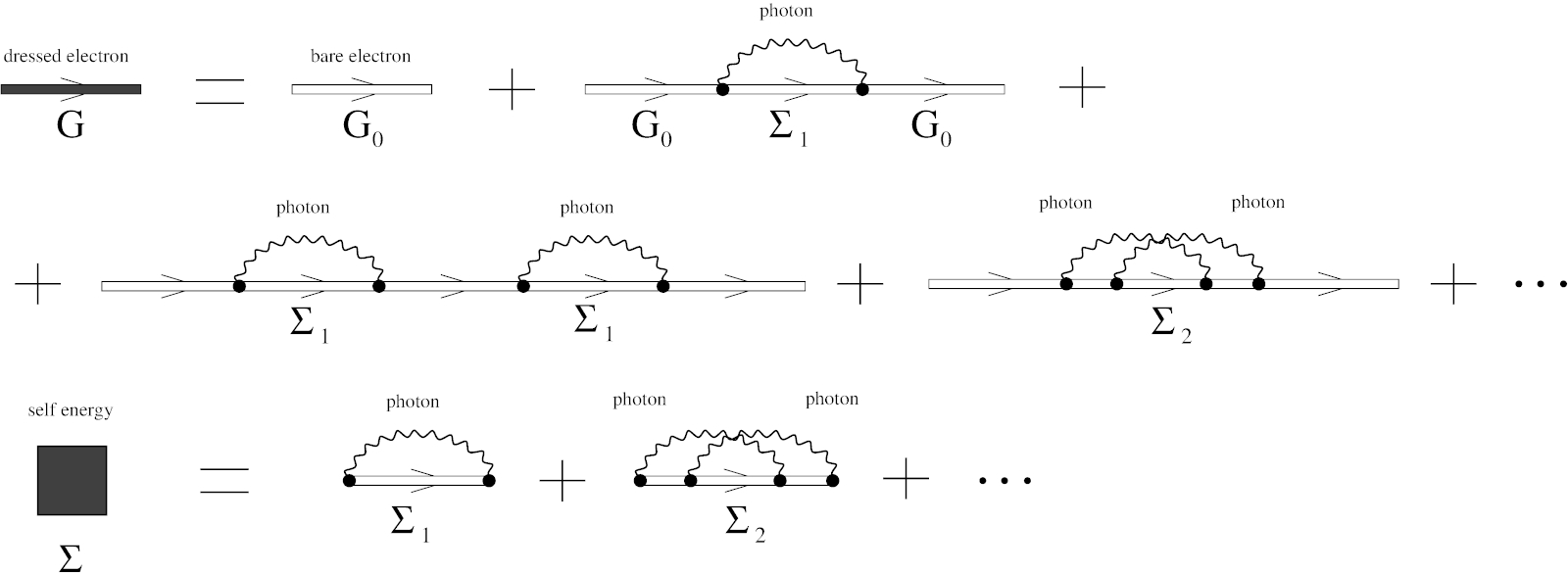Figure 5: The single-electron Green's function $$G$$ and the self-energy operator $$\Sigma$$.

The Dyson equation for the electron Green's function can be schematically written as following (compare it to Fig. 5) \begin{equation}\tag{21} G = G_0 + G_0 \cdot \Sigma \cdot G_0 + G_0 \cdot \Sigma \cdot G_0 \cdot \Sigma \cdot G_0 + \cdots \end{equation} It should be noted that in the Dyson equation every “multiplication” operation is an integration, for example, the second term in Eq.(21) should be understood as \begin{equation}\tag{22} G_0 \cdot \Sigma \cdot G_0 = \iint G_0(x_f,x) \Sigma(x,y) G_0(y,x_i) d^4x d^4y, \end{equation} where $$G_0$$ is the “bare” electron Green's function and $$\Sigma$$ is the self-energy operator (see Fig. 5).

Similar to Eq.(3) we can rewrite the equation for the electron Green's function as \begin{equation}\tag{23} G = G_0 + G_0 \cdot \Sigma \cdot G \end{equation} or in the integral form \begin{equation}\tag{24} G(x_f,x_i) = G_0(x_f,x_i) + \iint G_0(x_f,x) \Sigma(x,y) G(y,x_i) d^4x d^4y. \end{equation} Finally, we write a formal solution of Eqs.(23) and (24) in the form similar to the Geometric series solution (4). Using operator notations (where $$I$$ is a unit operator and $$G_0 \Sigma$$ is an integral operator) we get \begin{equation}\tag{25} (I - G_0 \Sigma) G = G_0 \end{equation} and, introducing inverse operators, the electron Green's function can be finally written as \begin{equation}\tag{26} G = (I - G_0 \Sigma)^{-1} G_0 = \frac{1}{I - G_0 \Sigma} G_0, \end{equation} where $$(I - G_0 \Sigma)^{-1}$$ is the inverse operator for $$(I - G_0 \Sigma)$$. Eq.(26) helps to analyze general properties of the electron Green's function, for example, it gives us a way to find poles of the electron Green's function (where the denominator $$(I - G_0 \Sigma)$$ becomes zero), which is important to determine the observable electron mass. We stop our consideration at this point. You probably agree that it is quite remarkable that a simple geometric series can manifest itself in quantum field theory equations.

As the last “hw” problem, could you try to proof the following identity? Note that working with operators is similar to working with matrices where the order of matrices is important ($$A\cdot B \ne B\cdot A$$). \begin{equation}\tag{27} G = \frac{1}{I - G_0 \Sigma} G_0 = \frac{1}{G_0^{-1} - \Sigma}, \end{equation} here $$G_0^{-1}$$ is the inverse operator for the electron Green's function $$G_0$$.